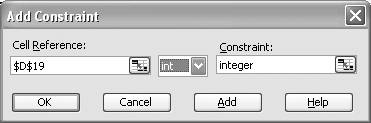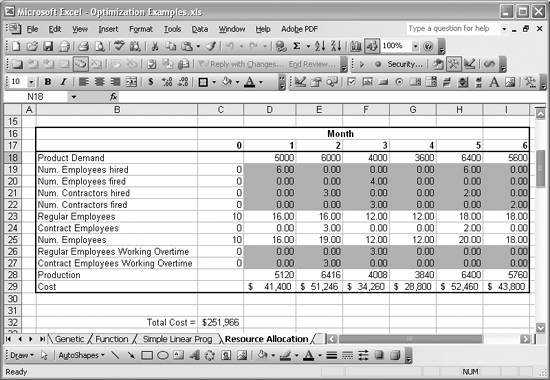# Getting More Realistic Results with Integer Constraints

Problem

You've performed an optimization problem where several variables were real valued, but realistically, certain values should only be integers. For example, in the previous recipe, the number of optimum lab technicians for a given month was 5.62. Obviously hiring 0.62 of a person would be hard (unless you're assuming the number represents part-time employment, in which case you could assume that 0.62 represents 62% of a regular 40-hour work week). Therefore, you want to constrain certain variables to be whole integers like 5 or 6.

Solution

Use Solver as discussed in the previous recipes, along with integer constraints on certain variables.

Discussion

Let's pick up where we left off with the resource allocation example from the previous recipe. What you need to do now is add several integer constraints. In fact, you want to require every value contained in cells D19 to I22 and D26 to I27 to assume only integer values. Real numbers will not be allowed.

You can add an integer constraint in Solver just as you would an ordinary constraint (see Recipe 9.4 for a discussion of constraints). After opening the Solver window, click the Add button to open the Add Constraint window. Select the cell you want to constrain and then select "int" from the drop-down list in the middle of the window, as shown in Figure 13-7.

Figure 13-7. Adding an integer constraintSolver will automatically enter "integer" in the Constraint field. Press Add for each constraint you want to add. A faster approach involves selecting an entire cell range in the Cell Reference field. For example, you could select the range D19:I22 to constrain all of the cells within that range to integer values. This approach avoids the tedium of having to add constraints one at a time for each cell in the range.

After you've added integer constraints, you can rerun Solver for this problem. Be prepared to wait, though. Adding integer constraints results in far greater computation time for this model. Whereas the first example (without integer constraints) converged on a solution in a matter of seconds, this second example (with integer constraints) took several hours to find a solution. In fact, it was taking so long on my computer that I left it running overnight, since I couldn't stay awake waiting for Solver to finish.

When Solver did finish, it yielded the results presented in Figure 13-8.

Figure 13-8. Resource allocation results with integer constraintsIn this case, the minimum total cost comes to \$251,966. This is a little higher than the earlier results discussed in the previous recipe. The reason is that Solver had to round up the number of employees hired or fired, and so on. Therefore, you end up with a little excess labor capacity. The production numbers reflect this excess capacity, where you can see that production will exceed demand by a little bit each month.

A further refinement of this problem would involve carrying over the excess production from one month to the next and factoring it in the required labor optimization each month. I'll leave that exercise to you.Excel Scientific and Engineering Cookbook (Cookbooks (OReilly))
ISBN: 0596008791
EAN: 2147483647
Year: N/A
Pages: 206
Authors: David M Bourg